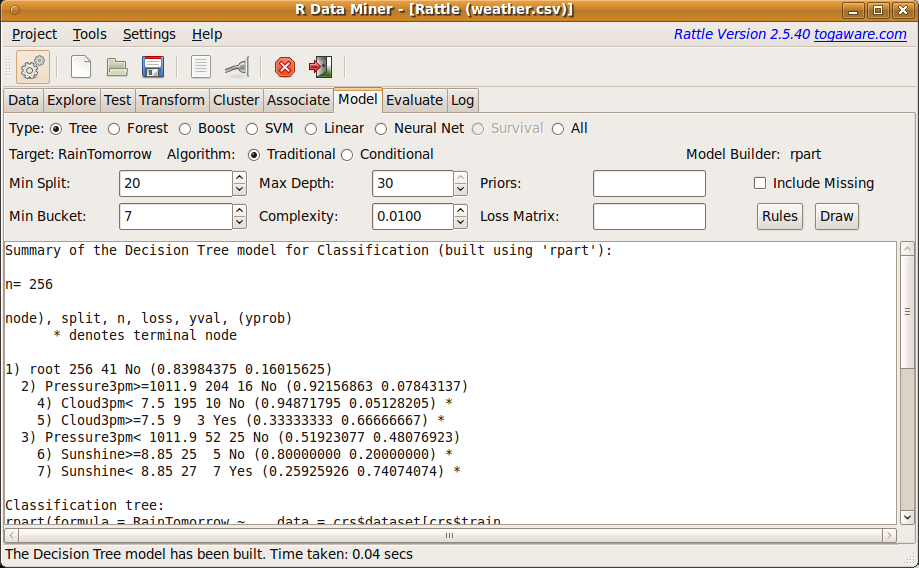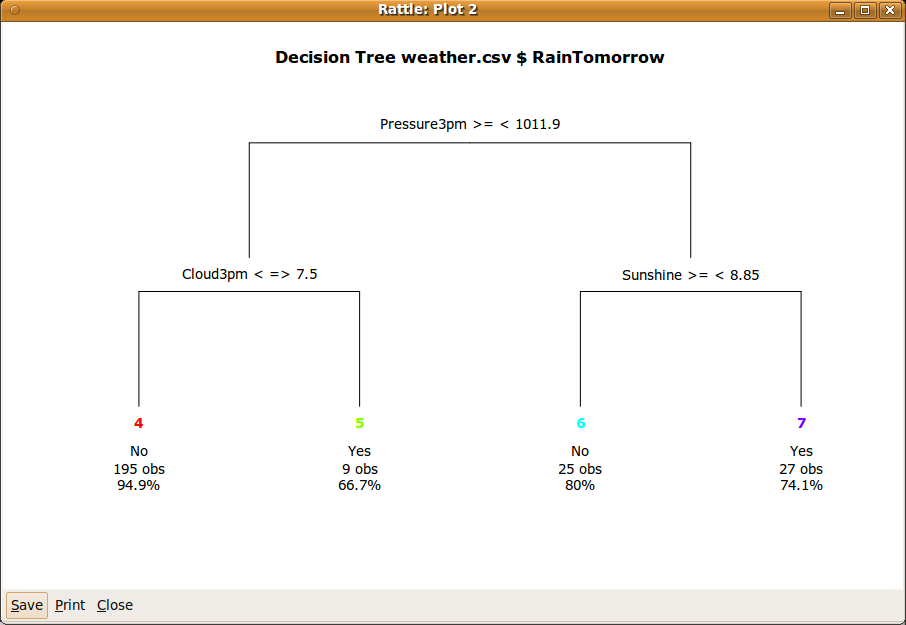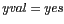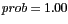DATA MINING
Desktop Survival Guide
by Graham Williams# Building a Model

Using Rattle we click the Model tab to be presented with the Model options (Figure 2.4). To build a decision tree model, one of the most common data mining models, click the Execute button. A textual representation of the model is shown in Figure 2.4.The target variable is RainTomorrow, as we would see if we were to scroll the Data tab window in Figure 2.3. Using the weather dataset our modelling task is to learn about the prospects of it raining tomorrow, given what we know about today. The model can be viewed in the R Console using the print command (the reference crs\$rpart identifies where the model itself has been saved, and the parameter Roption[]digits specifies the precision of the printed numbers).

We click in the R Console to make it active and type the following print command at the prompt (the prompt is the > character). The command itself consists of the name of an R function we wish to call upon (print in this case), followed by a list of arguments we pass to the function. The arguments provide information about what we want the function to do. After typing the full command (including the function name and arguments) we press the Enter key to pass the command to R. R will respond with the text exactly as we below, which starts with an indication of number of observations (256), followed by a textual presentation of the model:

 ```> print(crs\$rpart, digits=1) ```

 ```n= 256 node), split, n, loss, yval, (yprob) * denotes terminal node 1) root 256 40 No (0.84 0.16) 2) Pressure3pm>=1e+03 204 20 No (0.92 0.08) 4) Cloud3pm< 8 195 10 No (0.95 0.05) * 5) Cloud3pm>=8 9 3 Yes (0.33 0.67) * 3) Pressure3pm< 1e+03 52 20 No (0.52 0.48) 6) Sunshine>=9 25 5 No (0.80 0.20) * 7) Sunshine< 9 27 7 Yes (0.26 0.74) * ```

This textual presentation (which can also be seen in Figure 2.4) will take a little effort to understand and is further explained in Chapter 20. For now we might click on the Draw button provided by Rattle, to obtain the plot that we see in Figure 2.5. The plot provides a better idea of why it is called a decision tree.Clicking the Rules button will display a list of rules that are derived directly from the decision tree (we'll need to scroll the panel contained in the model tab to see them). The rules are listed here, and we explain them in detail, below.

 ``` Rule number: 7 [yval=Yes cover=27 (11%) prob=0.74] Pressure3pm< 1012 Sunshine< 8.85 Rule number: 5 [yval=Yes cover=9 (4%) prob=0.67] Pressure3pm>=1012 Cloud3pm>=7.5 Rule number: 6 [yval=No cover=25 (10%) prob=0.20] Pressure3pm< 1012 Sunshine>=8.85 Rule number: 4 [yval=No cover=195 (76%) prob=0.05] Pressure3pm>=1012 Cloud3pm< 7.5 ```

A well-recognised advantage of the decision tree representation for a model is that the paths through the decision tree can be interpreted as a collection of rules, as we have just seen. The rules are perhaps somewhat more readable. They are listed in the order of the probabily that is listed with each rule. The interpretation of the probability will be explained in Chpater. Rule number 23 (which also corresponds to the 23 in Figure 2.4 and node number 23 in Figure 2.5) is the strongest rule predicting rain (having the highest probability). We can read it as saying that if the humidity at 3pm was less than 73.5%, and the atmospheric pressure (reduced to mean sea level) at 3pm was less than 1010 hectopascals, and the amount of sunshine today was less than 8.85 hours, and the wind direction at 9am was one of E, ENE, N, NNW, SE, SSE, or WSW, then it seems there is a pretty good chance of rain tomorrow (and). That is to say, every time we have seen these conditions in the past (as represented in the data) it has always rained the following day.

Progressing down to the other end of the list of rules, rule number 10 tells us that if, instead, the amount of sunshine is greater than or equal to 8.85 (but with the same humidity and pressure conditions at 3pm), then it is less likely to be raining tomorrow (in this case, it suggests only a 6% probability (prob=0.06).

We now have our first model. We have data mined our historic observations of weather, to help provide some insight about the likelihood of it raining tomorrow.